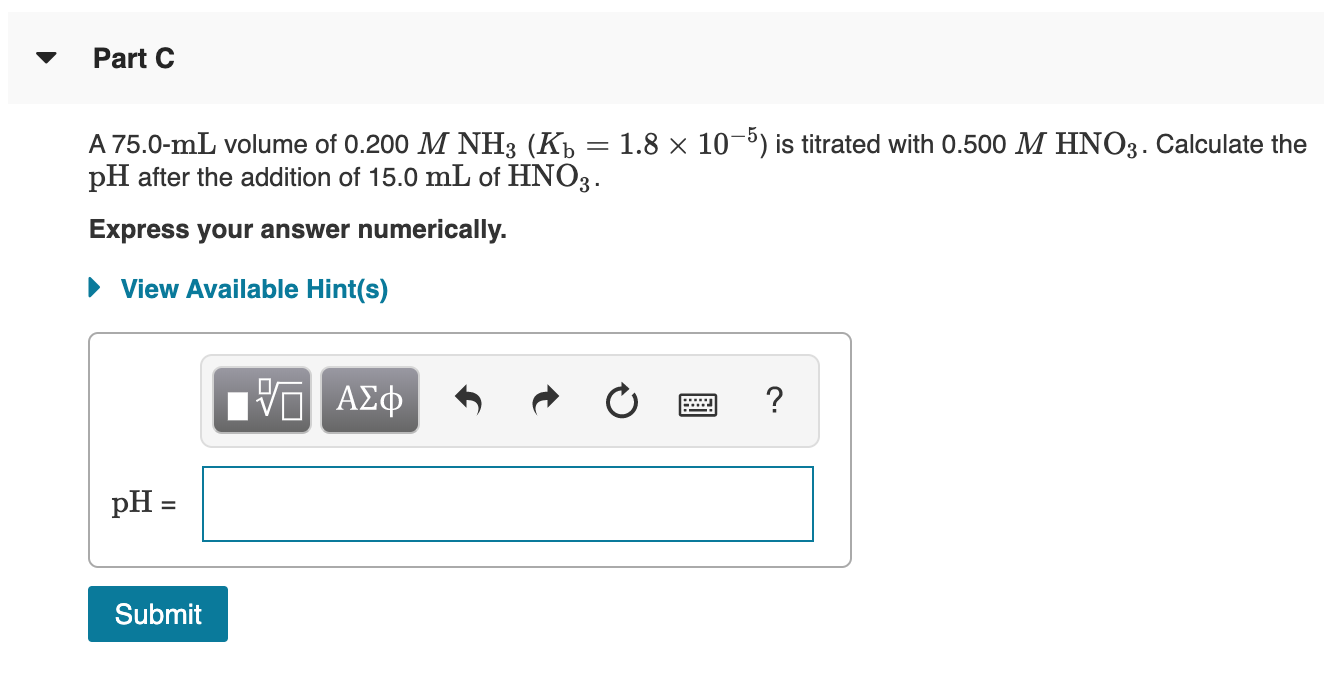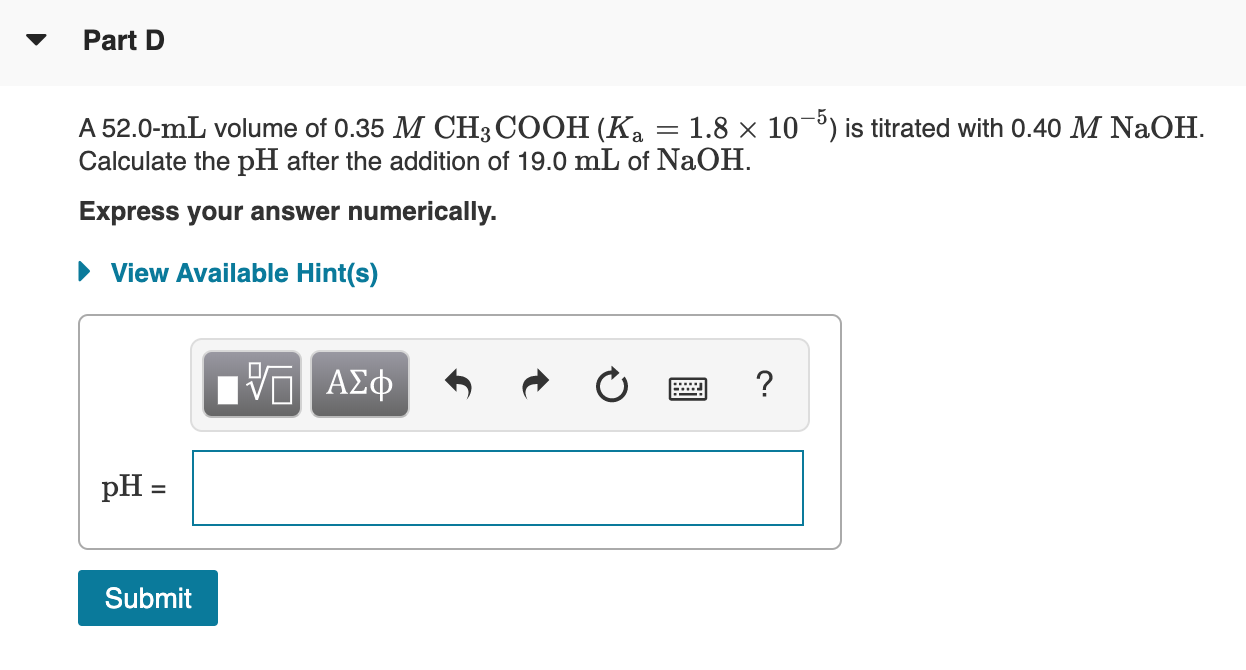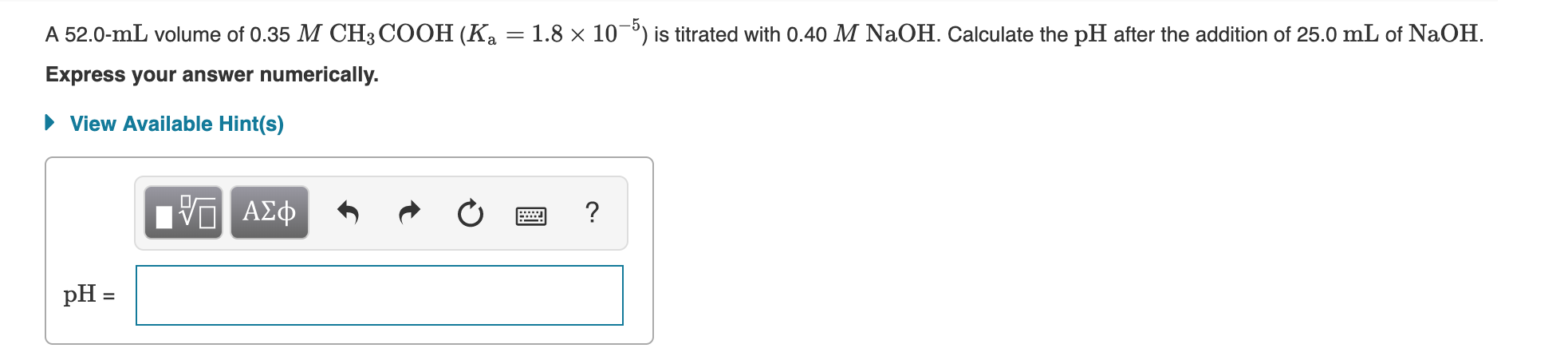# Part C A 75.0-mL volume of 0.200 M NH3 (K) = 1.8 x 10-5) is titrated...Part C A 75.0-mL volume of 0.200 M NH3 (K) = 1.8 x 10-5) is titrated with 0.500 M HNO3. Calculate the pH after the addition of 15.0 mL of HNO3 . Express your answer numerically. ► View Available Hint(s) O ALQ * R 0 2 ? pH = Submit
Part D A 52.0-mL volume of 0.35 M CH3COOH (Ka = 1.8 x 10-5) is titrated with 0.40 M NaOH. Calculate the pH after the addition of 19.0 mL of NaOH. Express your answer numerically. ► View Available Hint(s) TO AL N O a ? pH = Submit

C)
Given:
M(HNO3) = 0.5 M
V(HNO3) = 15 mL
M(NH3) = 0.2 M
V(NH3) = 75 mL

mol(HNO3) = M(HNO3) * V(HNO3)
mol(HNO3) = 0.5 M * 15 mL = 7.5 mmol

mol(NH3) = M(NH3) * V(NH3)
mol(NH3) = 0.2 M * 75 mL = 15 mmol

We have:
mol(HNO3) = 7.5 mmol
mol(NH3) = 15 mmol

7.5 mmol of both will react
excess NH3 remaining = 7.5 mmol
Volume of Solution = 15 + 75 = 90 mL
[NH3] = 7.5 mmol/90 mL = 0.0833 M
[NH4+] = 7.5 mmol/90 mL = 0.0833 M

They form basic buffer
base is NH3
conjugate acid is NH4+

Kb = 1.8*10^-5

pKb = - log (Kb)
= - log(1.8*10^-5)
= 4.745

use:
pOH = pKb + log {[conjugate acid]/[base]}
= 4.745+ log {8.333*10^-2/8.333*10^-2}
= 4.745

use:
PH = 14 - pOH
= 14 - 4.7447
= 9.2553

D)
Given:
M(CH3COOH) = 0.35 M
V(CH3COOH) = 52 mL
M(NaOH) = 0.4 M
V(NaOH) = 19 mL

mol(CH3COOH) = M(CH3COOH) * V(CH3COOH)
mol(CH3COOH) = 0.35 M * 52 mL = 18.2 mmol

mol(NaOH) = M(NaOH) * V(NaOH)
mol(NaOH) = 0.4 M * 19 mL = 7.6 mmol

We have:
mol(CH3COOH) = 18.2 mmol
mol(NaOH) = 7.6 mmol

7.6 mmol of both will react

excess CH3COOH remaining = 10.6 mmol
Volume of Solution = 52 + 19 = 71 mL
[CH3COOH] = 10.6 mmol/71 mL = 0.1493M

[CH3COO-] = 7.6/71 = 0.107M

They form acidic buffer
acid is CH3COOH
conjugate base is CH3COO-

Ka = 1.8*10^-5

pKa = - log (Ka)
= - log(1.8*10^-5)
= 4.745

use:
pH = pKa + log {[conjugate base]/[acid]}
= 4.745+ log {0.107/0.1493}
= 4.6

##### Add Answer of: Part C A 75.0-mL volume of 0.200 M NH3 (K) = 1.8 x 10-5) is titrated...
Similar Homework Help Questions
• ### Titrations Part A A 75.0-mL volume of 0.200 M NH3 (Kb=1.8×10−5) is titrated with 0.500 M HNO3. Calculate the pH after...

Titrations Part A A 75.0-mL volume of 0.200 M NH3 (Kb=1.8×10−5) is titrated with 0.500 M HNO3. Calculate the pH after the addition of 19.0 mL of HNO3. Express your answer numerically. Part B A 52.0-mL volume of 0.35 M CH3COOH (Ka=1.8×10−5) is titrated with 0.40 M NaOH. Calculate the pH after the addition of 23.0 mL of NaOH. Express your answer numerically.

• ### A 52.0-mL volume of 0.35 M CH3COOH (Ka = 1.8 x 10-5) is titrated with 0.40...A 52.0-mL volume of 0.35 M CH3COOH (Ka = 1.8 x 10-5) is titrated with 0.40 M NaOH. Calculate the pH after the addition of 25.0 mL of NaOH. Express your answer numerically. ► View Available Hint(s) O AQ 0 2 ? pH = A 75.0-mL volume of 0.200 M NH3 (Kb = 1.8 x 10-5) is titrated with 0.500 M HNO3. Calculate the pH after the addition of 28.0 mL of HNO3. Express your answer numerically. ► View Available...

• ### A 75.0-mL volume of 0.200 M NH3 (Kb=1.8�10?5) is titrated with 0.500 M HNO3. Calculate the pH after the addition of 11...

A 75.0-mL volume of 0.200 M NH3 (Kb=1.8�10?5) is titrated with 0.500 M HNO3. Calculate the pH after the addition of 11.0mL of HNO3. A 52.0-mL volume of 0.35 M CH3COOH (Ka=1.8�10?5) is titrated with 0.40 M NaOH. Calculate the pH after the addition of 31.0mL of NaOH.

• ### A 50.0-mL volume of 0.15 M HBr is titrated with 0.25 M KOH. Calculate the pH...

A 50.0-mL volume of 0.15 M HBr is titrated with 0.25 M KOH. Calculate the pH after the addition of 12.0 mL of KOH. Express your answer numerically. A 75.0-mL volume of 0.200 M NH3 (Kb=1.8×10−5) is titrated with 0.500 M HNO3. Calculate the pH after the addition of 13.0 mL of HNO3. Express your answer numerically. A 52.0-mL volume of 0.35 M CH3COOH (Ka=1.8×10−5) is titrated with 0.40 M NaOH. Calculate the pH after the addition of 33.0 mL...

• ### A 50.0-mL volume of 0.15 M HBr is titrated with 0.25 M KOH. Calculate the pH after the addition of 15.0 mL of KOH. Exp...

A 50.0-mL volume of 0.15 M HBr is titrated with 0.25 M KOH. Calculate the pH after the addition of 15.0 mL of KOH. Express your answer numerically. pH = SubmitHintsMy AnswersGive UpReview Part Part C A 75.0-mL volume of 0.200 M NH3 (Kb=1.8×10−5) is titrated with 0.500 M HNO3. Calculate the pH after the addition of 27.0 mL of HNO3. Express your answer numerically. pH = SubmitHintsMy AnswersGive UpReview Part Part D A 52.0-mL volume of 0.35 M CH3COOH...

• ### 1) A 50.0-mL volume of 0.15 M HBr is titrated with 0.25 M KOH. Calculate the pH after the addition of 16.0 mL of KOH....

1) A 50.0-mL volume of 0.15 M HBr is titrated with 0.25 M KOH. Calculate the pH after the addition of 16.0 mL of KOH. Express your answer numerically.   pH=????????? 2) A 75.0-mL volume of 0.200 M NH3 ( Kb = 1.8 x10-5) is titrated with 0.500 M HNO3. Calculate the pH after the addition of 13.0 mL of HNO3. Express your answer numerically.   pH=????????? 3) A 52.0-mL volume of 0.35 M CH3COOH ( Ka = 1.8 x10-5 ) is...

• ### A 75.0-mL volume of 0.200 M NH3 (Kb=1.8×10?5) is titrated with 0.500 M HNO3. Calculate the pH after the addition of 28....

A 75.0-mL volume of 0.200 M NH3 (Kb=1.8×10?5) is titrated with 0.500 M HNO3. Calculate the pH after the addition of 28.0 mL of HNO3. Express your answer numerically.

• ### ? Part D A 30.0-mL volume of 0.50 M CH3COOH (Ka 1.8 x 10-5) was titrated with 0.50 M NaOH. Calculate the pH af...

? Part D A 30.0-mL volume of 0.50 M CH3COOH (Ka 1.8 x 10-5) was titrated with 0.50 M NaOH. Calculate the pH after addition of 30.0 mL of NaOH at 25 C Express the pH numerically View Available Hint(s) PH Submit Return to Assigment Provide Feedback

• ### A 52.0-mL volume of 0.35 M CH3COOH (Ka=1.8×10−5 ) is titrated with 0.40 M NaOH . Calculate the pH after the addition o...

A 52.0-mL volume of 0.35 M CH3COOH (Ka=1.8×10−5 ) is titrated with 0.40 M NaOH . Calculate the pH after the addition of 15.0 mL of NaOH . Express your answer numerically.

• ### A 75.0-mL volume of 0.200 M NH3 (Kb=1.8×10−5) is titrated with 0.500 M HNO3. Calculate the pH after the addition of 13.0...

A 75.0-mL volume of 0.200 M NH3 (Kb=1.8×10−5) is titrated with 0.500 M HNO3. Calculate the pH after the addition of 13.0 mL of H

Need Online Homework Help?